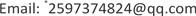1. 引言

2. 实验方案

E = F / A n / L = 4 mgL πd 2 n

3. 数据处理

F = [ f ( 0 , 0 ) f ( 0 , 1 ) … f ( 0 , n − 1 ) f ( 1 , 0 ) f ( 1 , 1 ) … f ( 1 , n − 1 ) ⋮ ⋮ ⋮ ⋮ f ( m − 1 , 0 ) f ( m − 1 , 1 ) … f ( m − 1 , n − 1 ) ]

S = 2 m m s i z e ( 一 个 黑 色 部 分 的 宽 所 占 像 素 点 数 )

Wire extension pixel count () and actual elongation (L1
L1−7.770E-07−2.176E-054.429E-051.803E-04−1.057E-043.388E-04−1.919E-04−1.911E-043.955E-042.727E-04
−3.846E-02−1.077E+002.192E+008.923E+00−5.231E+001.677E+01−9.500E+00−9.462E+001.958E+011.350E+01

4. 结语